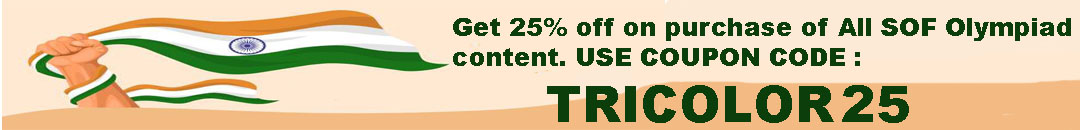# User ForumSubject :IMO    Class : Class 3

Subject :IMO    Class : Class 7

## Ans 1:

Class : Class 9
All fruits together = 100% OR x Apples = 30% of x OR 30/100 of x = 3/10 x Oranges= 50% of (x - 3/10 x) OR 1/2 of (x - 3/10 x) = 1/2 of 7/10 x = 7/20 x Pears = 20/20(All Fruits) - {6/20(Apples) + 7/20(Oranges)} = 20/20 - 13/20 = 7/20 Therefore it is clear that 7/20 of all fruits is pears. Hope this was helpful!

## Ans 2:

Class : Class 7

Subject :IMO    Class : Class 3

Subject :IMO    Class : Class 6

Subject :IMO    Class : Class 8

Subject :IMO    Class : Class 8

Subject :IMO    Class : Class 8

Subject :IMO    Class : Class 4

Class : Class 6
Thanks

## Ans 2:

Class : Class 6
I do not understand this question. Someone please can you help me.

## Ans 3:

Class : Class 5
Perimeter of 1st figure = 10+7+6+5+4+12 = 44 cm In second figure two opposite sides make 7+7 = 14 cm So, remaining two sides = 44 - 14 = 30 cm So, one side is 30/2 = 15 cm

Subject :IMO    Class : Class 7

## Ans 1:

Class : Class 8
pythagors theorum = 5*2 +12*2 =c*2 c=13 m height broken 5m height = 13m + 5m =18 m